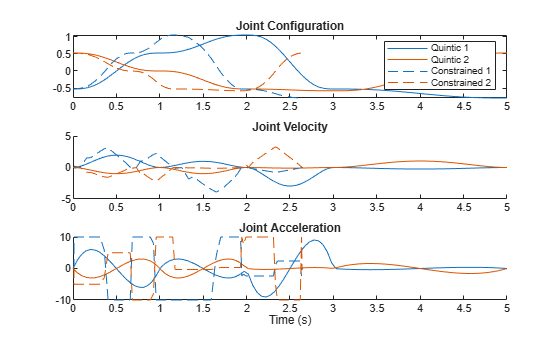# Generate Time-Optimal Trajectories with Constraints Using TOPP-RA Solver

This example shows how to generate trajectories that satisfy velocity and acceleration limits. The example uses the `contopptraj` function, which solves a constrained time-optimal path parametrized (TOPP) trajectory using reachability analysis (RA).

### Background

This example solves a TOPP problem, which is a robotics problem in which the goal is to find the fastest way to traverse a path subject to system constraints. In this example, you use the `contopptraj` function which solves a TOPP problem subject to velocity and acceleration constraints by using a method based on reachability analysis (RA), coined TOPP-RA . Other methods to solve TOPP problems rely on numerical integration (NI) or convex optimization (CO). Applications of TOPP include:

• Re-timing a joint-space trajectory for a manipulator to meet manufacturer-provided kinematic constraints.

• Generating a joint-space trajectory that returns fast and optimal trajectories given a planned path.

• Optimizing the traversal of a mobile robot path in SE(3) given limits on its motion.

You can use the `contopptraj` function in these ways:

• Generate a trajectory that connects waypoints while satisfying velocity and acceleration limits. In this case, use a minimum jerk trajectory as the initial guess. For more information, see Create Kinematically Constrained Trajectory.

• Re-paramterize an existing trajectory while preserving its timing given velocity and acceleration constraints. The path, which consists of waypoints in the N-dimensional input space, is a trajectory of any kind. The `contopptraj` function then scales how the robot navigates the path in the same time, mapping the existing trajectory to a new one that solves the TOPP problem.

In this example, you update an existing trajectory that connects five 2-D waypoints. The initial path is interpolated with an initial trajectory based on a quintic polynomial, which provides the shape. You then use the `contopptraj` function to apply velocity and acceleration limits to the initial path.

### Define Waypoints and Initial Trajectory

Both trajectories connect the same set of waypoints, subject to a set of velocity and acceleration limits. Specify the waypoints.

`waypts = (deg2rad(30))*[-1 1 2 -1 -1.5; 1 0 -1 -1.1 1];`

Different trajectories have different geometric and kinematic attributes, which affects how they navigate a path in space. The Choose Trajectories for Manipulator Paths example illustrates some differences between the various trajectory functions provided in Robotics System Toolbox™.

For this example, use a quintic polynomial trajectory to connect the wayponts. The quintic polynomial connects segments by using smooth velocity and acceleration profiles.

```tpts = [0 1 2 3 5]; timeVec = linspace(tpts(1),tpts(end),100); [q1,qd1,qdd1,pp1] = quinticpolytraj(waypts,tpts,timeVec);```

### Refine Trajectory Using Constrained TOPP-RA Solver

The quintic polynomial produces an output that, with default parameters, stops at each waypoint. You can use a TOPP-RA solver to compute the fastest possible way to traverse the path while still stopping at each waypoint, given bounded velocity and acceleration. Use the `contopptraj` function to generate a trajectory that traverses the initial path as quickly as possible while satisfying the specified velocity and acceleration limits.

```vellim = [-40 40; -25 25]; accellim = [-10 10; -10 10]; [q2,qd2,qdd2,t2] = contopptraj(pp1,vellim,accellim,numsamples=100);```

Plot the initial and modified trajectory on the same plot. Notice how the TOPP-RA solver speeds up the trajectory while still satisfying the known constraints.

```% Create a figure and adjust the color order so that the second two lines % have the same color as the associated first two lines figure c = colororder("default"); c(3:4,:) = c(1:2,:); colororder(c) % Plot results subplot(3,1,1) plot(timeVec,q1); hold on plot(t2,q2,"--") legend("Quintic 1","Quintic 2","Constrained 1","Constrained 2") title("Joint Configuration") xlim([0 tpts(end)]) subplot(3,1,2) hold on plot(timeVec,qd1) plot(t2,qd2,"--") title("Joint Velocity") xlim([0 tpts(end)]) subplot(3,1,3) hold on plot(timeVec,qdd1) plot(t2,qdd2,"--") title("Joint Acceleration") xlabel("Time (s)") xlim([0 tpts(end)])```### References

1. Pham, Hung, and Quang-Cuong Pham. “A New Approach to Time-Optimal Path Parameterization Based on Reachability Analysis.” IEEE Transactions on Robotics, 34, no. 3 (June 2018): 645–59. https://doi.org/10.1109/TRO.2018.2819195.